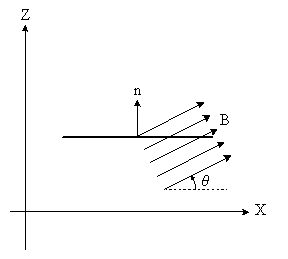# Magnetic Flux Question

Yosty22

## Homework Statement

A horizontal rectangular surface has dimensions 3.10cm by 3.05cm and is in a uniform magnetic field that is directed at an angle of 34.5∘ above the horizontal. What must the magnitude of the magnetic field be in order to produce a flux of 4.5E-4 Wb through the surface?

## Homework Equations

Magnetic Flux = BAcos(θ)

## The Attempt at a Solution

Using the above equation, I solved for B, getting B=Flux/Acos(θ)
I then plugged in my numbers: (4.5*10^-4)/((.0305)(.031)cos(34.5)) and got that B should equal 0.5775T. However, it says the answer is wrong, any ideas what I did wrong?

Mentor
I'd suggest that you make a sketch. You're looking for the flux through a horizontal surface, which means you want the vertical component of the B field...

Alternatively, take a vector equation approach and construct vectors for B and the area normal, then expand

##\Phi = \vec{B}\cdot (A\vec{n})##

Yosty22
So how do I go about finding that, though? I understand that I need the vertical component, but I have no idea how to set it up if all I know is the angle?

Mentor
So how do I go about finding that, though? I understand that I need the vertical component, but I have no idea how to set it up if all I know is the angle?

Make a sketch! Draw a horizontal line to show your area of interest in profile. Draw a vector or two representing the B field. What angle do you need? You can also choose the appropriate trig function and use the angle as given.You want the component of B that's parallel to the surface normal of your area.

#### Attachments

Yosty22
Okay, so I would say that B_n (normal component of the B field) = Bsin(34.5), then I can do the flux divided by sin(34.5) times the area, so I have B=4.5*10^-4/((sin34.5)(.031)(.0305)). That way I get B=0.84?

Mentor
Okay, so I would say that B_n (normal component of the B field) = Bsin(34.5), then I can do the flux divided by sin(34.5) times the area, so I have B=4.5*10^-4/((sin34.5)(.031)(.0305)). That way I get B=0.84?

Sure. Add the appropriate units and you're good.